# Problem: Consider this initial-rate data at a certain temperature for the reaction described byDetermine the rate law and the value of the rate constant for this reaction.rate = k  a) [OH-]b) [OH-]2c) [OCl-]d) [I-]e) [OCl-]2f) [I-]2k = ______

###### FREE Expert Solution

(a) To calculate the order of the reaction with respect to each reactant, we will use the experimental data given.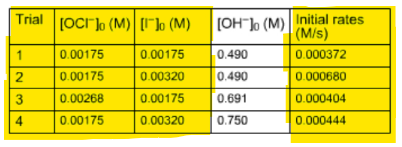OCl-

Look for the experimental data where the concentration of OCl- changes while the concentration of I- remains the same.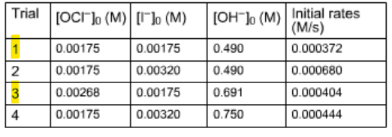Calculate order with respect to OCl-:

81% (38 ratings)###### Problem Details

Consider this initial-rate data at a certain temperature for the reaction described by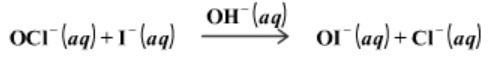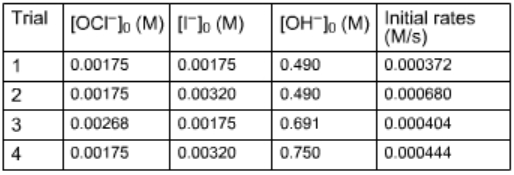Determine the rate law and the value of the rate constant for this reaction.

rate = k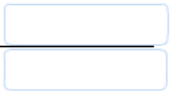a) [OH-]

b) [OH-]2

c) [OCl-]

d) [I-]

e) [OCl-]2

f) [I-]2

k = ______